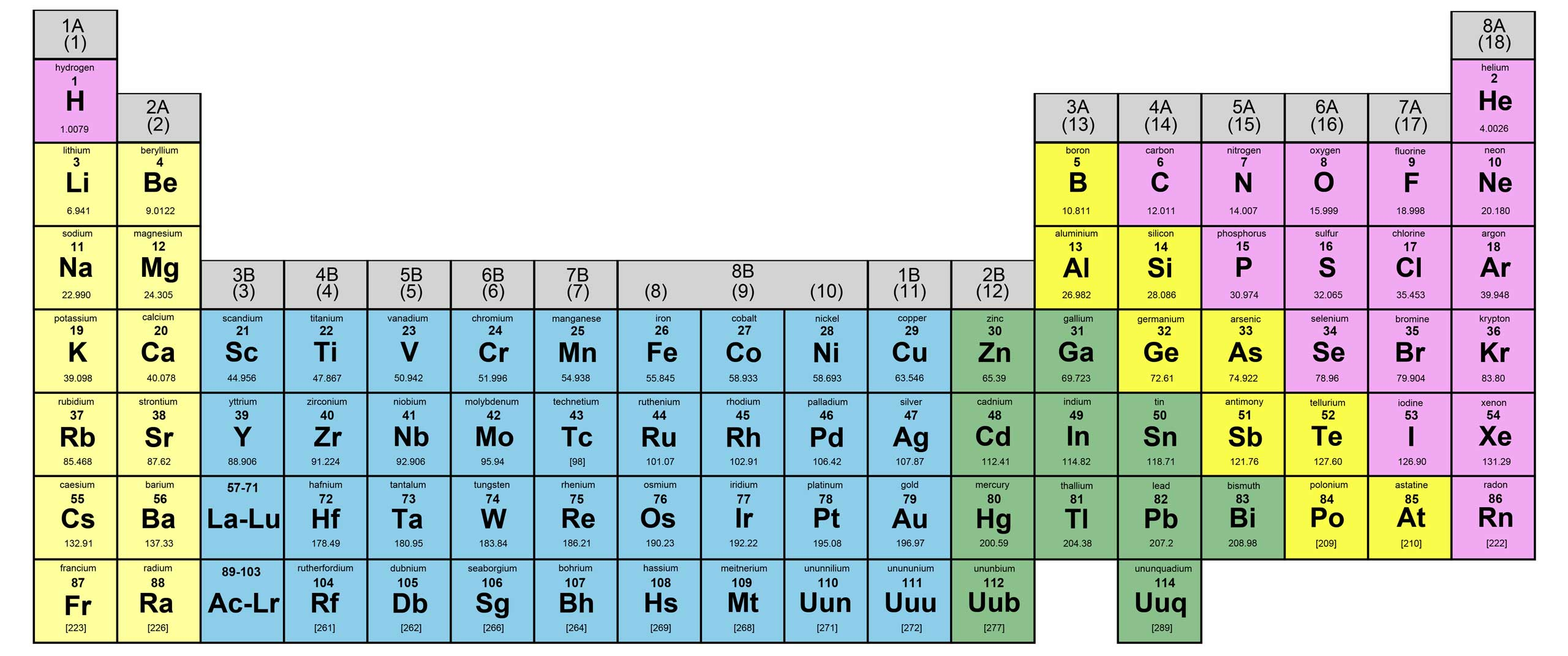Glossary:
 Magma A molten mass of rock material within the earth Magnetic Quantum Number The quantum number that is related to the orientation of the orbitals in space Main-group (representative) elements elements in the groups labeled I A, 2A, 3A, 4A, 5A, 6A, 7A, and 8A in the periodic table The group number gives the sum of valence s and p electrons Major species the components present in relatively large amounts in a solution Malleable Capable of being shaped by beating with a hammer or by rolling Manometer a device for measuring the pressure of a gas in a container Mantle The largest portion of the earth, located between the core and the crust Mass the quantity of matter in an object Mass defect the change in mass occurring when a nucleus is formed from its component nucleons Mass number the total number of protons and neutrons in the atomic nucleus of an atom Mass percent the percent by mass of a component of a mixture (11 1) or of a given element in a compound Mass spectrometer an instrument used to determine the relative masses of atoms by the deflection of their ions in a magnetic field Matter the material of the universe Maximum Work The work done by a systern during a thermodynarnically reversible change Meniscus The curved surface of a liquid at the liquid-air interface in a narrow tube Messenger RNA (mRNA) a special RNA molecule built in the cell nucleus that migrates into the cytoplasm and participates in protein synthesis Metal an element that gives up electrons relatively easily and is lustrous, malleable, and a good conductor of heat and electricity Metallic Bond The electrostatic attraction binding the positive ions of a solid metal together by means of a "sea" of delocalized valence electrons Metalloenzyme an enzyme containing a metal ion at its active site Metalloids (semimetals) elements along the division line in the periodic table between metals and nonmetals These elements exhibit both metallic and nonmetallic properties Metallurgy The techniques employed in obtaining a metal from its ore and preparing it for practical use Metamorphic Rocks Rocks formed from other rocks-igneous, sedimentary, or metamorphic-through the agency of extreme temperatures and pressures Micelles aggregates of fatty acid anions having their hydrophobic tails in the interior and their polar heads pointing outward to interact with the polar water molecules Minerals Substances present in the earth's crust having a uniform composition and a definite crystalline structure Miscible Capable of mixing in all proportions to form a homogeneous mixture Mixture a material of variable composition that contains two or more substances Model (theory) a set of assumptions put forth to explain the observed behavior of matter. The models of chemistry usually involve assumptions about the behavior of individual Moderator a substance used in a nuclear reactor to slow down the neutrons Molal boiling-point elevation constant a constant characteristic of a particular solvent that gives the change in boiling point as a function of solution molality; used in molecular weight determinations Molal freezing-point depression constant a constant characteristic of a particular solvent that gives the change in freezing point as a function of the solution molality; used in motecular weight determinations Molality the number of moles of solute per kilogram of solvent in a solution Molar Absorptivity The proportionality constant in the Beer-Lambert law Molar heat capacity the energy required to raise the temperature of one mole of a substance by one degree Celsius Molar mass the mass in grams of one mole of molecules or formula units of a substance; also called molecular weight Molar volume the volume of one mole of an ideal gas; equal to 22.42 liters at STP Molarity moles of solute per volume of solution in liters Mole (mol) the number equal to the number of carbon atoms in exactly 12 grams of pure '2C: Avogadro's number One mole represents 6022 X 1023 units Mole fraction the ratio of the number of moles of a given component in a mixture to the total number of moles in the mixture Mole ratio (stoichiometry) the ratio of moles of one substance to moles of another substance in a balanced chemical equation Molecular equation an equation representing a reaction in solution showing the reactants and products in undissociated form, whether they are strong or weak electrolytes Molecular formula the exact formula of a molecule, giving the types of atoms and the number of each type Molecular orbital (MO) model a model that regards a molecule as a collection of nuclei and electrons, where the electrons are assumed to occupy orbitals much as they do in atoms, but having the orbitals extend over the entire molecule In this model the electrons are assumed to be delocalized rather than always located between a given pair of atoms Molecular orientations (kinetics) orientations of molecules during collisions, some of which can lead to reaction while others cannot Molecular solid a solid composed of neutral molecules at the lattice points Molecular structure the three-dimensional arrangement of atoms in a molecule Molecular weight the mass in grams of one mole of molecules or formula units of a substance; the same as molar mass Molecularity the number of species that must collide to produce the reaction represented by an elementary step in a reaction mechanism Monochromatic Referring to radiant energy of a single wavelength Monodentate Ligand a ligand that can form one bond to a metal ion Monoprotic acid an acid with one acidic proton Monosaccharide (simple sugar) a polyhydroxy ketone or aldehyde containing from three to nine carbon atoms Myoglobin an oxygen-storing biomolecule consisting of a heme complex and a proteinnews | about us | contact us tutorials index | organic chemistry | practice tests | online quizzes | reference tools site copyright (c) 2002-2013 Learn Chem